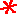Main      Site Guide### Scrambled Equations

• Scrambled Equations

In each of these puzzles, you are given a number that you must construct out of several other numbers. You do this by taking the numbers and performing addition, subtraction, multiplication, and/or division operations on them. Each number must be used in the calculations exactly once, and only these four operations listed may be used. You may parenthesize your expression however you wish. For example, 5 may be obtained from 1, 2, and 3, with the expression (3 + 2) × 1.

In many if not most cases, multiple solutions are possible, but usually only one is given on the solution page.

## #76

Make 31 out of 1, 6, 8, and 9.

Hint

Solution

## #77

Make 46 out of 2, 3, 8, and 8.

Hint

Solution

## #78

Make 24 out of 4, 4, 7, and 7.

Hint

Solution

## #79Make 24 out of 1, 5, 5, and 5.

Hint

Solution

## #80Make 24 out of 3, 3, 8, and 8.

Hint

Solution

## #81

Make 100 out of 1, 3, 4, and 8.

Hint

Solution

## #82

Make 99 out of 1, 3, 4, and 8.

Hint

Solution

## #83

Make 52 out of 4, 6, 7, and 8.

Hint

Solution

## #84

Make 51 out of 1, 2, 6, and 9.

Hint

Solution

## #85

Make 8 out of 3, 3, 3, and 3.

Hint

Solution

## #86

Make 90 out of 2, 4, 4, and 5.

Hint

Solution

## #87

Make 16 out of 1, 3, 6, and 9.

Hint

Solution

## #88

Make 36 out of 2, 2, 3, and 7.

Hint

Solution

## #89

Make 38 out of 2, 2, 3, and 7.

Hint

Solution

## #90

Make 106 out of 2, 6, 6, and 9.

Hint

Solution

## #91

Make 45 out of 2, 7, 7, and 8.

Hint

Solution

## #92

Make 43 out of 4, 4, 5, and 6.

Hint

Solution

## #93

Make 100 out of 1, 3, 5, and 7.

Hint

Solution

## #94

Make 25 out of 1, 2, 2, and 9.

Hint

Solution

## #95

Make 37 out of 3, 5, 8, and 9.

Hint

Solution

## #96

Make 4 out of 2, 7, 7, and 9.

Hint

Solution

## #97

Make 76 out of 6, 7, 7, and 8.

Hint

Solution

## #98

Make 7 out of 6, 7, 7, and 8.

Hint

Solution

## #99

Make 20 out of 1, 1, 2, and 7.

Hint

Solution

## #100Make 48 out of 1, 6, 7, and 8.

Hint

Solution